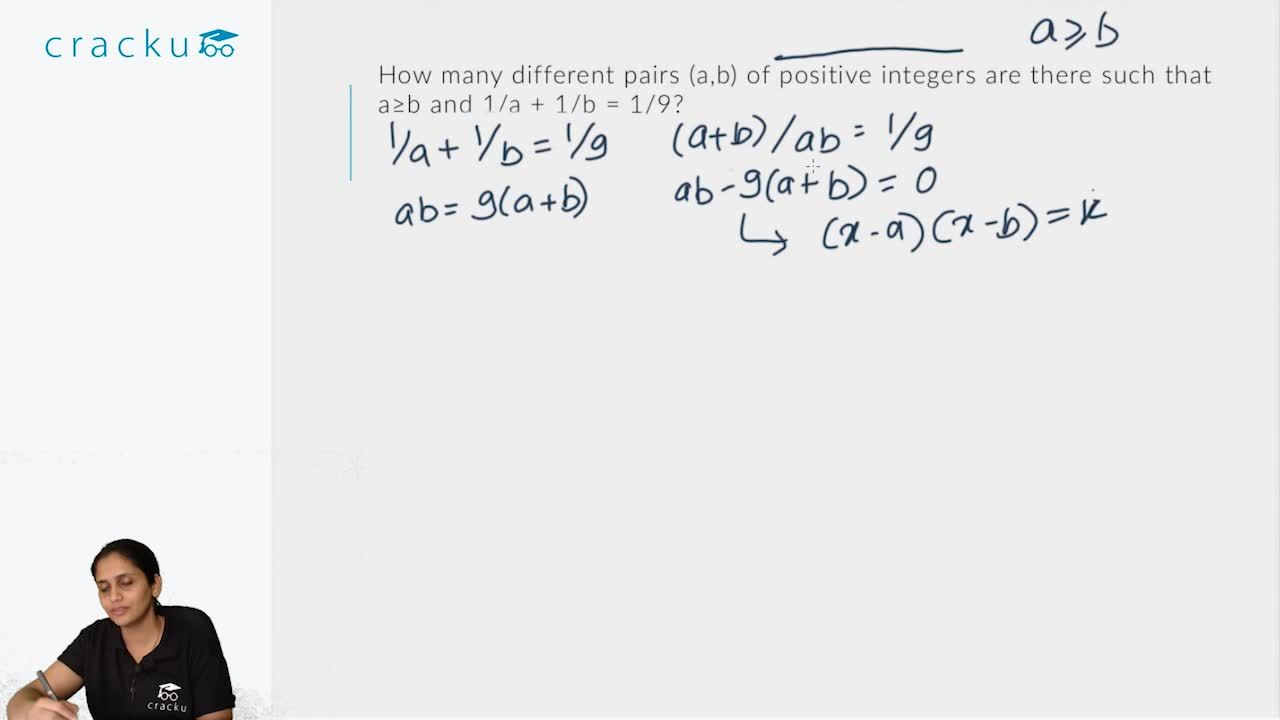Question 28

# How many different pairs(a,b) of positive integers are there such that $$a\geq b$$ and $$\frac{1}{a}+\frac{1}{b}=\frac{1}{9}$$?

Solution

$$\frac{1}{a}+\frac{1}{b}=\frac{1}{9}$$
=> $$ab = 9(a + b)$$
=> $$ab - 9(a+b) = 0$$
=> $$ab - 9(a+b) + 81 = 81$$
=> $$(a - 9)(b - 9) = 81, a > b$$
Hence we have the following cases,
$$a - 9 = 81, b - 9 = 1$$ => $$(a,b) = (90,10)$$
$$a - 9 = 27, b - 9 = 3$$ => $$(a,b) = (36,12)$$
$$a - 9 = 9, b - 9 = 9$$ => $$(a,b) = (18,18)$$
Hence there are three possible positive integral values of (a,b)

### View Video Solution##### Atvee

2 weeks ago

I don't know why people are saying they don't understand her explanation. I can follow along even when I am playing all these in 2x speed.
Anyone familiar with cat algebra should be able to so.
And her explanation in the full revision videos for quant in the cracku youtube channel was top notch. (Thanks a lot maam).
Watch that and come back or get a good understanding of the concepts, all your complaints will end.

##### Palash Jamnekar

2 weeks ago

i literally skit the que..coz of this lady....coz of her poor teaching style...(ahh ahh ahhh) hi bol deri 10 bar toh bich bich me

##### rahul

8 months, 1 week ago

couldn't understand the solution